## Classification

#### Classification

Direction: In each problem, out of the five figures marked (a), (b), (c), (d) and (e), four are similar in a certain manner. However one figure is not like the other four. Choose the figure which is different from the rest.

1. Choose the figure which is different from the rest.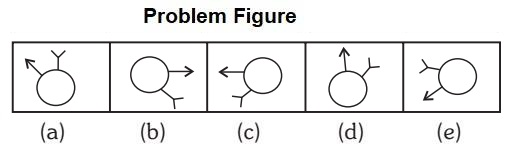1. All other figures can be rotated into each other.

##### Correct Option: C

All other figures can be rotated into each other.

1. In following questions, select the one which is different from the other three responses.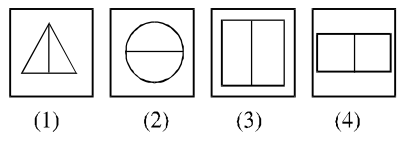1. Except one figure, all others the design has been divided vertically.

##### Correct Option: B

Except in figure (2), in all others the design has been divided vertically.

1. In the following question, find the odd one out from the given alternatives.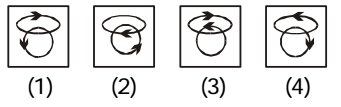1. The arrow points in different direction in figure (4).

##### Correct Option: D

The arrow points in different direction in figure (4). But all other figures are in same direction.

1. In the following questions find the odd figures from the given responses.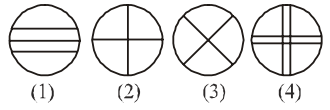1. Except one figure, all others the circle has been divided into four equal parts.

##### Correct Option: A

Except in figure (1), in all others the circle has been divided into four equal parts.

1. In the following questions find the odd figure from the given responses.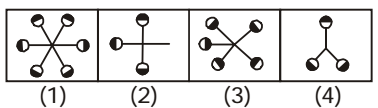1. In all other figures, the components of design are placed at equi-distance. Again, the shading pattern of circle is different in figure (3).

##### Correct Option: C

Except in figure (3), in all other figures, the components of design are placed at equi-distance. Again, the shading pattern of circle is different in figure (3).